Taking regular meter readings is still required on many different types of business energy meters. If meter readings are not sent to your energy supplier, then you could risk getting inaccurate energy bills.

Get a quick quote and save up to 46%

To make sure that you always get accurate energy bills you should be sending regular meter readings to your energy supplier. If you have a half-hourly meter or a smart meter then don’t worry, your supplier will automatically get sent this information.

## How to read my electricity meter

Electricity meters will mostly have a digital screen showing the meter reading. With digital meters, you could have a single-rate meter or a two-rate meter. If you had your meter fitted quite a while ago it could be a dial meter, we will explain the difference below.

### Single rate meters

Single rate meters will show five numbers followed by a red number. To read the meter write down the numbers from left to right. Ignore any numbers after the first time.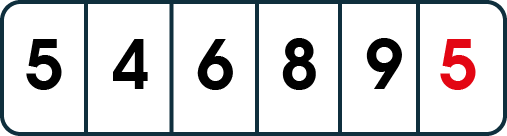This meter reading would show 54689

### Two rate meters

Depending on what type of meter you have you could have two separate meter readings. If you have separate unit rates for day and night you most probably have an economy 7 or 10 meters.

One set of numbers will be labelled low or night as this shows how many units of cheaper electricity has been used. The other set will be labelled normal or day. This will record how much electricity you have used at the standard rate.

Read the numbers in the same way you would a single rate meter.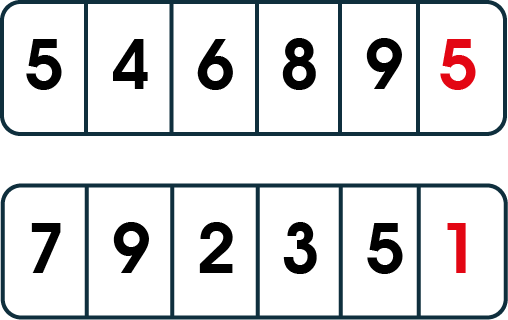This meter reading would be 54689 and 79235

## Dial meters

Electricity dial meters usually have five or more dials. You only need to focus on the first five to get your reading. You want to read the numbers from left to right.

If the dial pointer is between two numbers then write down the lower number, unless it is between zero and nine in this case were down nine. If the pointer is directly on a number then underline this number. If the dial next to the underlined number is between zero and nine, then take one away from the underlined number.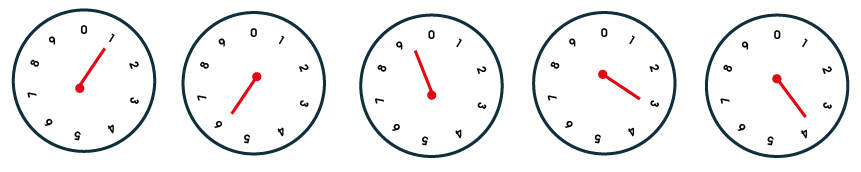## How to read my gas meter

Like electricity meters, you could either have a digital gas meter or a dial meter. If you have a digital meter then it could be a metric meter or an imperial meter. You will be able to tell the difference as a metric meter will have five main numbers and an imperial meter will have four.

### Metric meter

With a metric meter, you will have a row of numbers. Read the first five numbers from left to right. When writing down the numbers you should include any zeros at the beginning. Don’t include any numbers after a space or the decimal point. A metric meter will start recording again from zero once it has reached 99,999.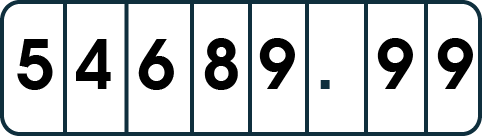This meter reading would be 54689

### Imperial meter

For an imperial gas meter, you should also take the reading from left to right. You should write down all the numbers including zeros. Don’t include any of the numbers in red.This meter reading would be 5468

## Dial meter

Older gas meters will most likely have a dial reading instead of a row of numbers. With a dial meter, you need to read the numbers from left to right, just as you would with a digital meter.  Sometimes the pointer can be between two numbers, always choose the lower number. Unless the pointer is between zero and nine, in this case, write down nine.

If any of the pointers are exactly on a number, then underline it. If you have any nines written down, then take one away from the underlined number.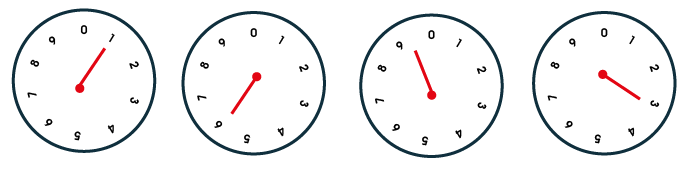This meter reading would be 1593